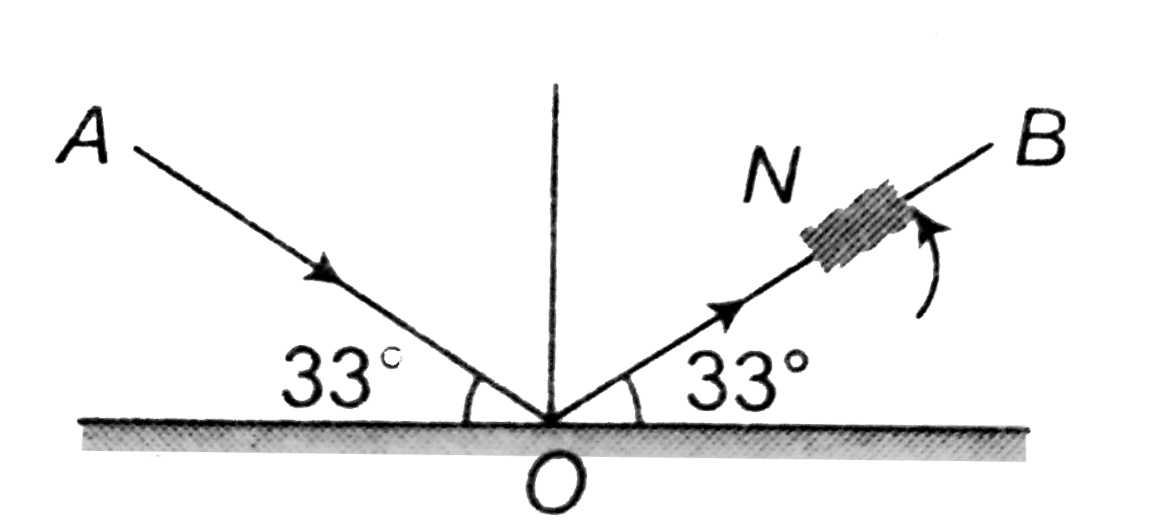# A beam of light AO is incident on a glass slab (mu=1.54) in a direction as shown in figure. The reflected ray OB is passed throught a Nicol pris

28 views
in Physics
closed
A beam of light AO is incident on a glass slab (mu=1.54) in a direction as shown in figure. The reflected ray OB is passed throught a Nicol prism. On viewinf through a Nicole prism, we find rotating the prism that (tan^(-1)1.54=57^(@))A. the intensity is reduced down to zero and remains zero
B. the intensity reduces down some what and rises again
C. there is no change in intensity
D. the intensity gradually reduces to zero and then again increases

by (84.0k points)
selected

Angle of polarisation p=tan^(-1)(mu)=tan^(-1)(1.54)=57^(@)
i=p=57^(@), reflected light is completely polarisd